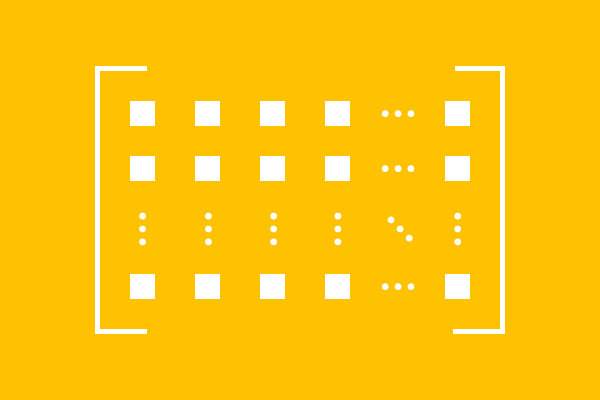# Types of matrices

The entries (or elements) are arranged in rows and columns for expressing them in matrix form. The arrangement possibly forms either a rectangle and square. Hence, the matrices are basically classified into two types.

1. Square matrix
2. Rectangular matrix

Let’s start to learn each type of matrix with understandable examples.

### Square matrixThe arrangement of elements in a case forms a square shape in a matrix.

${\begin{bmatrix} 2 & 9 & 1 & 3 \\ 7 & 1 & 0 & 5 \\ 6 & 8 & 2 & 6 \\ 0 & 6 & 3 & 5 \\ \end{bmatrix}}$

It is possible if the number of elements in a row is exactly equal to the number of elements in a column. Hence, the matrix is called a square matrix.

### Rectangular matrixThe arrangement of elements in the remaining case forms a rectangle shape in a matrix.

${\begin{bmatrix} 8 & 0 & 4 & 2 \\ 6 & 2 & 3 & 8 \\ 7 & 4 & 5 & 1 \\ \end{bmatrix}}$

It is possible when the number of elements in a row is not equal to the number of elements in a column. Hence, the matrix is called a rectangular matrix.

In mathematics, there are two types of rectangular matrices. Let’s learn both types of rectangular matrices with some understandable examples.

Latest Math Topics
Jun 26, 2023
Jun 23, 2023

Latest Math Problems
Jul 01, 2023
Jun 25, 2023
###### Math Questions

The math problems with solutions to learn how to solve a problem.

Learn solutions

Practice now

###### Math Videos

The math videos tutorials with visual graphics to learn every concept.

Watch now

###### Subscribe us

Get the latest math updates from the Math Doubts by subscribing us.